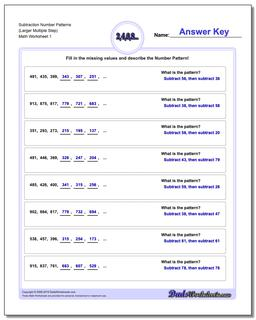# Math Worksheets: Number Patterns: Number Patterns: Subtraction Number Patterns (Larger Multiple Step)## Subtraction Number Patterns (Larger Multiple Step)

PropertyValue
DescriptionSubtraction Number Patterns (Larger Multiple Step): Number pattern problems using only subtraction operations.
Resource TypeWorksheet